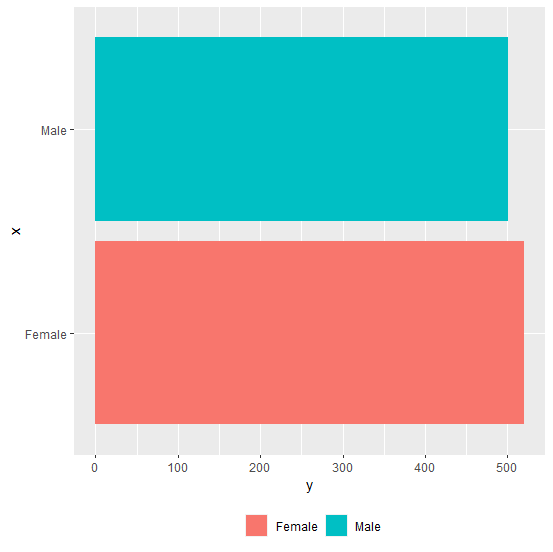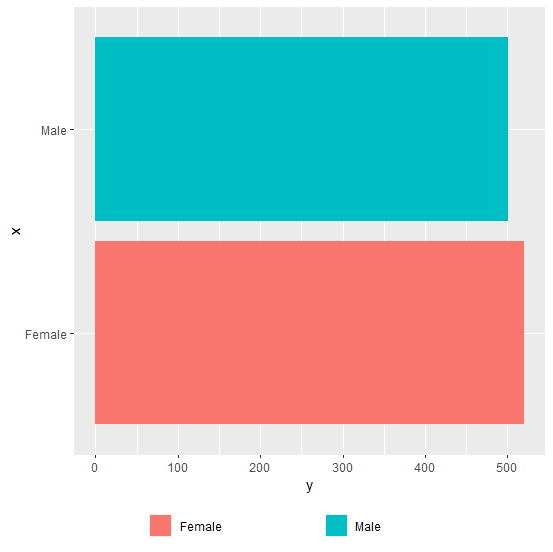# How to increase the space between horizontal legends using ggplot2 in R?

Generally, the space between two legend entries is not large enough and it becomes difficult to read the legend names if the names are long. In this case, we need to increase the margin between the legend entries/names but this would be required when the legends are horizontally aligned as vertical legends can be read as it is. For this purpose, we can use legend.text argument inside theme function of ggplot2 package.

## Example

Consider the below data frame −

Live Demo

> x<-c("Male","Female")
> y<-c(501,520)
> df<-data.frame(x,y)
> df

## Output

    x     y
1 Male   501
2 Female 520

## Example

> library(ggplot2)
> ggplot(df,aes(x,y,fill=x))+geom_bar(stat="identity")+coord_flip()+theme(legend.position="bottom")+guides(fill=guide_legend(title=NULL))

## OutputCreating the plot with a larger space between legend entries −

## Example

> ggplot(df,aes(x,y,fill=x))+geom_bar(stat="identity")+coord_flip()+theme(legend.position="bottom",legend.text=element_text(margin=margin(r=1,unit="inch")))+guides(fill=guide_legend(title=NULL))

## OutputUpdated on: 02-Jan-2021

372 Views